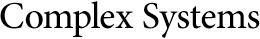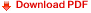## On Dynamical Properties of Neural NetworksFernanda Botelho
Max Garzon
Department of Mathematical Sciences and
Institute for Intelligent Systems,
Memphis State University, Memphis, TN 38152, USA

#### Abstract

A transform is introduced that maps cellular automata and discrete neural networks to dynamical systems on the unit interval. This transform is a topological conjugacy except at countably many points. In many cases, it gives rise to continuous full conjugates, in which case the transform preserves entropy. The transform also allows transfer of many dynamical properties of continuous systems to a large class of infinite discrete neural networks (including cellular automata). For instance, it is proved that the network dynamics of very simple classes of neural networks, even with highly symmetric architectures, have chaotic regions of evolution (in the sense of existence of scrambled sets and configurations of arbitrarily large periods). These results raise the possibility of fully modeling parallel computability on real-valued dynamical systems by discrete neural networks.